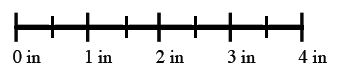### Home > ACC6 > Chapter 6 Unit 6 > Lesson CC1: 6.1.3 > Problem6-33

6-33.

Use a ruler to draw a line exactly $4$ inches long and then mark every $\frac { 1 } { 2 }$ inch.

1. How many $\frac { 1 } { 2 }$ inches are in $4$ inches?2. Now use the ruler to mark every $\frac { 1 } { 4 }$ inch. How many $\frac { 1 } { 4 }$ inches are in $1$ inch?

$\frac{1}{4}(?)=1$

3. How many $\frac { 1 } { 4 }$ inches are in $2$ inches? in $3$ inches?

• There are five fifths in $1$, ten fifths in $2$, and fifteen fifths in $3$.